CAT  >  Practice Questions Level 2: Mensuration - 2

# Practice Questions Level 2: Mensuration - 2 - Notes | Study Level-wise Practice Questions for CAT Preparation - CAT

 1 Crore+ students have signed up on EduRev. Have you?

This EduRev document offers 20 Multiple Choice Questions (MCQs) from the topic Mensuration (Level - 2). These questions are of Level - 2 difficulty and will assist you in the preparation of CAT & other MBA exams. You can practice/attempt these CAT Multiple Choice Questions (MCQs) and check the explanations for a better understanding of the topic.

Question for Practice Questions Level 2: Mensuration - 2
Try yourself:In the given diagram, a rope is wound around a circular drum whose diameter is 70 cm and a bucket is tied to the other end of the rope. Find the number of revolutions made by the drum if the bucket is to be raised by 11 m.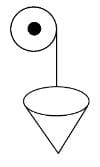Question for Practice Questions Level 2: Mensuration - 2
Try yourself:One of the base edges of a metallic plate, which is in the form of a right-angled triangle, is soldered to a wire. The wire is rotated around its own axis. What is the maximum volume of the shape obtained by rotating the metallic plate, given that the smallest side of the triangular plate is 8 cm in length and the largest side is 2 cm greater than the second largest side?

Question for Practice Questions Level 2: Mensuration - 2
Try yourself:A cylindrical vessel of diameter 14 cm and height 42 cm is fixed symmetrically inside a similar vessel of diameter 16 cm and height 42 cm. If the total space between the two vessels is filled with cork dust for heat insulation purposes, then how many cubic centimetres of cork dust will be required?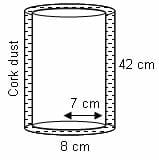Question for Practice Questions Level 2: Mensuration - 2
Try yourself:A tap drips at an average rate of 2 drops in 3 seconds. It takes 2080 drops of water to completely fill a hemispherical vessel of radius 8.3 cm. How many times can the vessel be filled in this manner in 13 hours?

Question for Practice Questions Level 2: Mensuration - 2
Try yourself:Ratio between the lateral surface area and the total surface area of a right circular cylinder is 3 : 5. If the lateral surface area is 1848 sq. m, then volume of the cylinder is (in cubic m).

Question for Practice Questions Level 2: Mensuration - 2
Try yourself:The given rectangle ABCD has length 9 and width 5. Diagonal AC is divided into 5 equal parts at W, X, Y and Z. Find the area of the shaded region.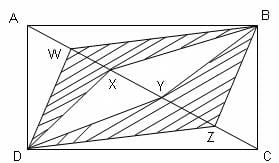Question for Practice Questions Level 2: Mensuration - 2
Try yourself:If 350 stones of spherical shape are dropped into a hemispherical cup containing some water, they get fully submerged. Diameter of the cup is 14 m. What will be the radius of the stone, if the water just spills off from the hemispherical cup?

Question for Practice Questions Level 2: Mensuration - 2
Try yourself:Find the approximate time taken to fill a chemical conical vessel, if the flow rate is 20 meters per minute from a cylindrical pipe whose diameter is 5 meters. Take diameter and depth of the conical vessel as 80 meters and 48 meters, respectively.

Question for Practice Questions Level 2: Mensuration - 2
Try yourself:A rectangular reservoir has dimensions 54 meters × 44 meters × 10 meters. An outlet pipe of circular cross-section has a radius of 3 centimeters and the water runs through the pipe at a rate of 20 meters/second. What is the time taken by the outlet pipe to empty the reservoir full of water?

Question for Practice Questions Level 2: Mensuration - 2
Try yourself:Two cubes, each of edge length 6 cm, are joined end to end. The surface area of the resulting cuboid is

Question for Practice Questions Level 2: Mensuration - 2
Try yourself:What is the volume (in cubic cm) of given figure?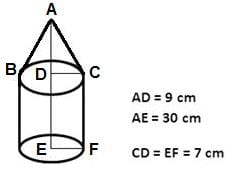Question for Practice Questions Level 2: Mensuration - 2
Try yourself:Consider a cylinder of height h cm and radius r = 2/π cm, as shown in the figure (not drawn to scale). A string of a certain length, when bound on its cylindrical surface starting at point A and ending at point B, gives a maximum of n turns (in other words, the string's length is the minimum length required to wind n turns). What is the vertical spacing in cm between the two consecutive turns?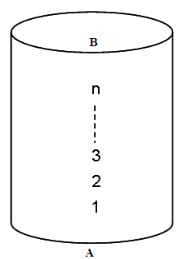Question for Practice Questions Level 2: Mensuration - 2
Try yourself:A solid is in the form of a cylinder with hemispherical ends. The total height of the solid is 19 cm and the diameter of the cylinder is 7 cm. The surface area of the solid is

Question for Practice Questions Level 2: Mensuration - 2
Try yourself:A tent is in the form of a right circular cylinder surmounted by a cone. The diameter of the cylinder is 24 m. The height of the cylindrical portion is 11 m, while the vertex of the cone is 16 m above the ground. What is the area of the curved surface for the conical portion?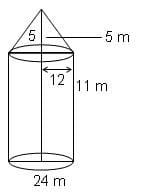Question for Practice Questions Level 2: Mensuration - 2
Try yourself:Let ℓ be the slant height of a frustum. If a and b represent the two base radii, which of the following expressions represents the ratio of the total surface area to the curved surface area of the frustum?

Question for Practice Questions Level 2: Mensuration - 2
Try yourself:Three spherical marbles are placed in a hollow inverted cone, one above the other. Each marble is in contact with the adjacent marble(s). Also, each marble is in contact with the wall of the cone. The smallest marble has a radius of 8 cm. The largest marble has a radius of 18 cm.

The radius of the middle marble is

Question for Practice Questions Level 2: Mensuration - 2
Try yourself:Increasing the height of a cone by 9 units increases its volume by x cubic units. Increasing its radius by 6 units also increases its volume by x cubic units. If the original height is 3 units, then the original radius is _____.

Question for Practice Questions Level 2: Mensuration - 2
Try yourself:A sealed bottle, which contains water, has been constructed by attaching a cylinder of radius 1 cm to a cylinder of radius 3 cm, as shown in Figure A. When the bottle is upright, the height of the water is 20 cm, as shown in the cross section of the bottle in Figure B. When the bottle is upside down, the height of the liquid is 28 cm, as shown in Figure C. What is the total height (in cm) of the bottle?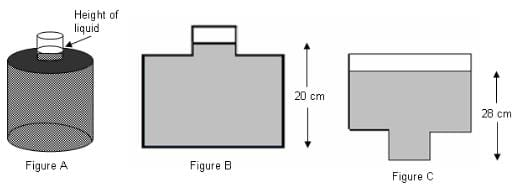Question for Practice Questions Level 2: Mensuration - 2
Try yourself:A certain pyramid has a square base with side 1 cm and four lateral faces, each of which is an equilateral triangle. A bug sitting at point X wishes to crawl over the faces of the pyramid to reach point C. Assuming that point X is half way up the pyramid, what is the length of the shortest trip he can take?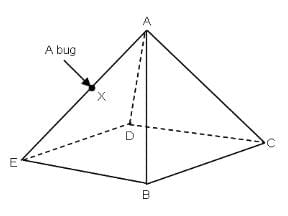Question for Practice Questions Level 2: Mensuration - 2
Try yourself:A cylindrical tank is 6 feet tall and its base is 4 feet in diameter. A bug crawls from a point P on the upper rim of the tank to a point Q which is directly 4 feet below P, going around the tank once in doing so. Then, the bug crawls from point Q to a point R, directly below P, on the bottom rim of the tank (the vertical distance from Q to R is 2 feet), again going around the can once in doing so. What is the length (in inches) of the shortest path that the bug could have taken from P to R?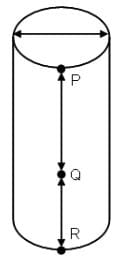The document Practice Questions Level 2: Mensuration - 2 - Notes | Study Level-wise Practice Questions for CAT Preparation - CAT is a part of the CAT Course Level-wise Practice Questions for CAT Preparation.
All you need of CAT at this link: CAT

## Level-wise Practice Questions for CAT Preparation

277 docs
 Use Code STAYHOME200 and get INR 200 additional OFF

## Level-wise Practice Questions for CAT Preparation

277 docs

### How to Prepare for CAT

Read our guide to prepare for CAT which is created by Toppers & the best Teachers

Track your progress, build streaks, highlight & save important lessons and more!

,

,

,

,

,

,

,

,

,

,

,

,

,

,

,

,

,

,

,

,

,

;